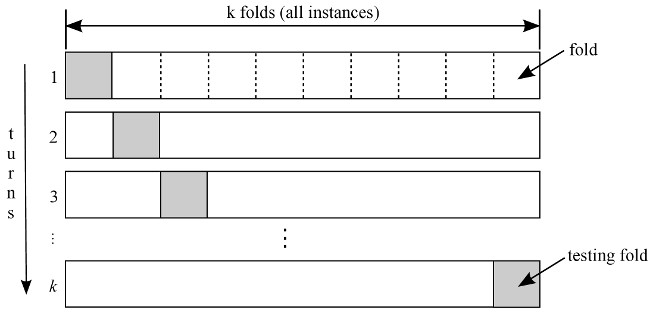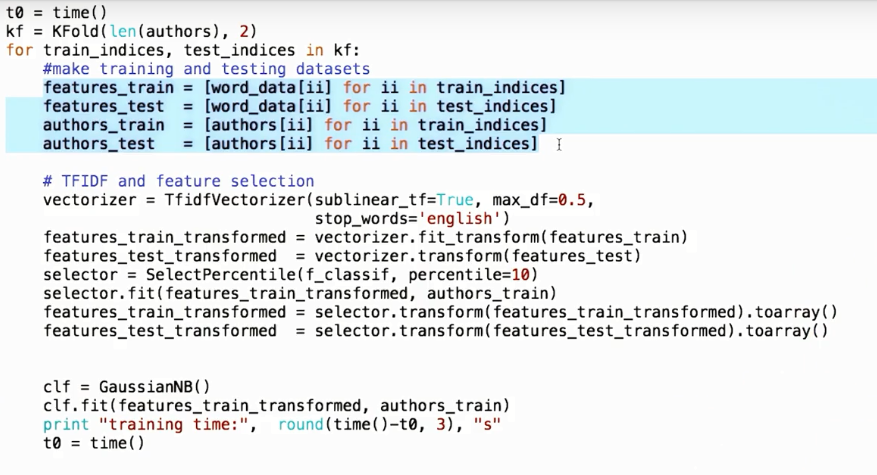2018-02-05 10:25:43 ChenVast 阅读数 6707
• ###### 机器学习&深度学习系统实战！

购买课程后，可扫码进入学习群，获取唐宇迪老师答疑 数学原理推导与案例实战紧密结合，由机器学习经典算法过度到深度学习的世界，结合深度学习两大主流框架Caffe与Tensorflow，选择经典项目实战人脸检测与验证码识别。原理推导，形象解读，案例实战缺一不可！具体课程内容涉及回归算法原理推导、决策树与随机森林、实战样本不均衡数据解决方案、支持向量机、Xgboost集成算法、神经网络基础、神经网络整体架构、卷积神经网络、深度学习框架--Tensorflow实战、案例实战--验证码识别、案例实战--人脸检测。 专属会员卡优惠链接：http://edu.csdn.net/lecturer/1079

39706 人正在学习 去看看 唐宇迪

holdout交叉验证K折交叉验证可以得到模型的泛化误差的可靠估计（模型在新数据集是的性能表现）。

## holdout交叉验证

holdout交叉验证是机器学习模型泛化性能的一个经典且常用的方法

holdout交叉验证能将最初的数据集分为训练集和测试集

评估模型应用于新数据上能够获得较小偏差

holdout过程：holdout缺点：模型性能评估对训练集划分为训练以及验证子集的方法是敏感的，评价的结果随样本的不同而发生变化

## K折交叉验证

K折交叉验证将k个训练子集重复holdout方法k次。

K折交叉验证不重复的随机将训练集划分为k个，k-1个用于训练，剩余一个用于测试，重复该过程k次，得到k个模型对模型性能的评价。

K折交叉验证使用了无重复抽样技术的好处：每次迭代过程中每个样本点只有一次被划入训练集或测试集的机会

K折交叉验证图：K折交叉验证的一个特例：

留一（LOO）交叉验证法：将数据子集划分的数量等于样本数（k=n），每次只有一个样本用于测试，数据集非常小时，建议用此方法。

K折交叉验证改进成的 分层K折交叉验证：

获得偏差和方差都低的评估结果，特别是类别比例相差较大时。

# 使用k-fold交叉验证来评估模型性能。
import numpy as np
from sklearn.cross_validation import StratifiedKFold

kfold = StratifiedKFold(y=y_train,
n_folds=10,
random_state=1)

scores = []
for k, (train, test) in enumerate(kfold):
pipe_lr.fit(X_train[train], y_train[train])
score = pipe_lr.score(X_train[test], y_train[test])
scores.append(score)
print('Fold: %s, Class dist.: %s, Acc: %.3f' % (k+1, np.bincount(y_train[train]), score))

print('\nCV accuracy: %.3f +/- %.3f' % (np.mean(scores), np.std(scores)))from sklearn.cross_validation import cross_val_score

scores = cross_val_score(estimator=pipe_lr,
X=X_train,
y=y_train,
cv=10,
n_jobs=1)
print('CV accuracy scores: %s' % scores)
print('CV accuracy: %.3f +/- %.3f' % (np.mean(scores), np.std(scores)))cross_val_score方法特点：将不同分块的性能评估分布到多个CPU上进行处理。

n_jobs：CPU个数，-1为选择全部CPU

k重交叉验证机器学习 相关内容

2019-01-30 16:30:56 XiaoYi_Eric 阅读数 504
• ###### 机器学习&深度学习系统实战！

购买课程后，可扫码进入学习群，获取唐宇迪老师答疑 数学原理推导与案例实战紧密结合，由机器学习经典算法过度到深度学习的世界，结合深度学习两大主流框架Caffe与Tensorflow，选择经典项目实战人脸检测与验证码识别。原理推导，形象解读，案例实战缺一不可！具体课程内容涉及回归算法原理推导、决策树与随机森林、实战样本不均衡数据解决方案、支持向量机、Xgboost集成算法、神经网络基础、神经网络整体架构、卷积神经网络、深度学习框架--Tensorflow实战、案例实战--验证码识别、案例实战--人脸检测。 专属会员卡优惠链接：http://edu.csdn.net/lecturer/1079

39706 人正在学习 去看看 唐宇迪

### 2.为什么用交叉验证？

• 交叉验证用在数据量不是很充足的情况(比如数据量小于一万条)，能够从有限的数据中获取尽可能多的有效信息。
• 交叉验证用于评估模型的预测性能，尤其是训练好的模型在新数据上的表现，能够一定程度上减小过拟合。

### 3.交叉验证方法

#### 3.1 留出法交叉验证

from sklearn import datasets
from sklearn import model_selection

#引入sklearn库中手写数字的数据集

#留出法
X_train, X_test, y_train, y_test = model_selection.train_test_split(digits.data, digits.target, test_size = 0.3, shuffle = True)


#### 3.2 k折交叉验证

k折交叉验证 (k-fold Cross Validation) 过程如下所示：

1. 不重复抽样将原始数据随机分成k份。

2. 每次挑选其中1份作为测试集，剩余k-1份作为训练集用于训练模型。

3. 重复第2步k次，在每个训练集上训练后得到一个模型。用这个模型在相应的测试集上测试，计算并保存模型的评估指标。

4. 计算k组测试结果的平均值作为模型准确度的估计，并作为当前k折交叉验证下模型的性能指标。k一般取10，数据量大的时候，k可以设置的小一些。数据量小的时候，k可以设置的大一些，这样训练集占整体数据的比例就比较大，不过同时训练的模型个数也就相应增加。

from sklearn import datasets
from sklearn import model_selection

#引入sklearn库中手写数字的数据集

#K折交叉验证
#设置K为5
kf = model_selection.KFold(n_splits=5)
#使用5折交叉验验证划分数据集，返回一个生成器对象（即索引）
digits_gen = kf.split(digits.data)
for train_idx, test_idx in digits_gen:
X_train = digits.data[train_idx] #训练集
X_test = digits.data[test_idx] #测试集
y_train = digits.target[train_idx] #训练集标签
y_test = digits.target[test_idx] #测试及标签


#### 3.3 留一法交叉验证

from sklearn import datasets
from sklearn import model_selection

#引入sklearn库中手写数字的数据集

#留一法交叉验证
loo = model_selection.LeaveOneOut()
digits_gen = loo.split(digits.data)
for train_idx, test_idx in digits_gen:
X_train = digits.data[train_idx]
X_test = digits.data[test_idx]
y_train = digits.target[train_idx]
y_test = digits.target[test_idx]


### 4.推广k重交叉验证机器学习 相关内容

2019-09-05 22:23:51 weixin_44791964 阅读数 724
• ###### 机器学习&深度学习系统实战！

购买课程后，可扫码进入学习群，获取唐宇迪老师答疑 数学原理推导与案例实战紧密结合，由机器学习经典算法过度到深度学习的世界，结合深度学习两大主流框架Caffe与Tensorflow，选择经典项目实战人脸检测与验证码识别。原理推导，形象解读，案例实战缺一不可！具体课程内容涉及回归算法原理推导、决策树与随机森林、实战样本不均衡数据解决方案、支持向量机、Xgboost集成算法、神经网络基础、神经网络整体架构、卷积神经网络、深度学习框架--Tensorflow实战、案例实战--验证码识别、案例实战--人脸检测。 专属会员卡优惠链接：http://edu.csdn.net/lecturer/1079

39706 人正在学习 去看看 唐宇迪

### 机器学习好伙伴之scikit-learn的使用——K折交叉验证# 什么是K折交叉验证

K折交叉验证首先要将整个数据集分成K份。
1、取前面K-1份用于训练，最后一份用于测试，并取得测试结果。
2、取前面K-2份和最后一份用于训练，取第K-1份用于测试，并取得测试结果。
3、以此类推，将数据集的每一份均用于测试过一次。
4、取所有测试结果取平均。# sklearn中K折交叉验证的实现

from sklearn.model_selection import cross_val_score


K折交叉验证的重要函数是：

cross_val_score(
estimator,
X, y=None,
scoring=None,
cv=None,
n_jobs=1,
verbose=0,
fit_params=None,
pre_dispatch=‘2*n_jobs’
)


1、estimator：用于预测的模型。
2、X：预测的特征数据
3、y：预测结果
4、scoring：调用的方法

https://scikit-learn.org/stable/modules/model_evaluation.html#scoring-parameter5、cv：交叉验证生成器或可迭代的次数

scores = cross_val_score(knn, X, y, cv=5, scoring='accuracy')


# 应用示例

from sklearn.datasets import load_iris
from sklearn.model_selection import train_test_split
# K折交叉验证模块
from sklearn.model_selection import cross_val_score
# 导入k聚类算法
from sklearn.neighbors import KNeighborsClassifier

# 加载iris数据集
X = iris.data
y = iris.target

# 分割数据
X_train, X_test, y_train, y_test = train_test_split(X, y, test_size = 0.3, random_state=4)

# 建立Knn模型
knn = KNeighborsClassifier()

# 使用K折交叉验证模块
scores = cross_val_score(knn, X, y, cv=5, scoring='accuracy')

# 将5次的预测准确率打印出
print(scores)

#将5次的预测准确平均率打印出
print(scores.mean())


[0.96666667 1.         0.93333333 0.96666667 1.        ]
0.9733333333333334


k重交叉验证机器学习 相关内容

2019-12-16 13:23:02 xiao_lxl 阅读数 14
• ###### 机器学习&深度学习系统实战！

购买课程后，可扫码进入学习群，获取唐宇迪老师答疑 数学原理推导与案例实战紧密结合，由机器学习经典算法过度到深度学习的世界，结合深度学习两大主流框架Caffe与Tensorflow，选择经典项目实战人脸检测与验证码识别。原理推导，形象解读，案例实战缺一不可！具体课程内容涉及回归算法原理推导、决策树与随机森林、实战样本不均衡数据解决方案、支持向量机、Xgboost集成算法、神经网络基础、神经网络整体架构、卷积神经网络、深度学习框架--Tensorflow实战、案例实战--验证码识别、案例实战--人脸检测。 专属会员卡优惠链接：http://edu.csdn.net/lecturer/1079

39706 人正在学习 去看看 唐宇迪

# sklearn中的K折交叉验证cv = KFold( len(authors), 2 )


cv = KFold( len(authors), 2, shuffle=True )


shuffle=True 可避免所有某一特定类型的事件都属于训练集，而另一特定类型的事件则属于测试集，在这种情况下，针对一种类型事件的训练不会帮助我们成功地对另一类型的事件进行分类

from sklearn.cross_validation import KFold
t0= time()
cv = KFold( len(authors), 2, shuffle=True ) #将 shuffle 标志设置为 true来随机化 Sklearn K-fold cross validation 中的事件
for train_indices,test_indices in kf:
# make training and testing datasets
features_train = [word_data[ii] for ii in train_indices] #利用索引进入特征和标签
features_test = [word_data[ii] for ii in test_indices]
authors_train = [authors[ii] for ii in train_indices]
authors_test = [authors[ii] for ii in train_indices]

# TFIDF and feature selection
vectorizer = TfidfVectorizer(sublinear_tf = True,max_df=0.5,
stop_words = 'english')
feature_train_transformed = vectorizer.fit_transform(features_train)
feature_test_transformed = vectorizer.transform(features_test)
selector = SelectPercentile(f_classif,percentile = 10)
selector.fit(features_train_transformed,authors_train)
features_train_transformed = selector.transform(features_train_transformed).toarray()
features_test_transformed = selector.transform(features_test_transformed).toarray()

clf = GaussianNB()
clf.fit(features_train_transformed,authors_train)
print "training time:" ,round(time() - t0,3),'s'
t0=time()
pred = clf.predict(features_test_transfomed)
print "predicting time",round(time() - t0,3),'s'
acc = accuracy_score(pred,authors_test)
print "accuracy:", round(acc,3)


# sklearn中的GridSearchCV

GridSearchCV 用于系统地遍历多种参数组合，通过交叉验证确定最佳效果参数。它的好处是，只需增加几行代码，就能遍历多种组合。

parameters = {'kernel':('linear', 'rbf'), 'C':[1, 10]}
svr = svm.SVC()
clf = grid_search.GridSearchCV(svr, parameters)
clf.fit(iris.data, iris.target)


parameters = {'kernel':('linear', 'rbf'), 'C':[1, 10]}


('rbf', 1)	('rbf', 10)
('linear', 1)	('linear', 10)


svr = svm.SVC()


clf = grid_search.GridSearchCV(svr, parameters)


clf.fit(iris.data, iris.target)


SVM 的哪些参数使用特征脸示例中的 GridSearchCV 进行自动调谐？
C和gamma

# 你的首个（过拟合）POI 标识符的准确度是多少？


import pickle
import sys
sys.path.append("../tools/")
from feature_format import featureFormat, targetFeatureSplit

sort_keys = '../tools/python2_lesson13_keys.pkl'

### first element is our labels, any added elements are predictor
### features. Keep this the same for the mini-project, but you'll
### have a different feature list when you do the final project.
features_list = ["poi", "salary"]

data = featureFormat(data_dict, features_list)
labels, features = targetFeatureSplit(data)

from sklearn import tree
clf = tree.DecisionTreeClassifier()
clf.fit(features, labels)
pred_dt = clf.predict(features)

from sklearn.metrics import accuracy_score
acc_dt = accuracy_score(pred_dt, labels)
print acc_dt  # 0.989473684211


# 适当部署的测试范围的准确度



import pickle
import sys
sys.path.append("../tools/")
from feature_format import featureFormat, targetFeatureSplit

sort_keys = '../tools/python2_lesson13_keys.pkl'

### first element is our labels, any added elements are predictor
### features. Keep this the same for the mini-project, but you'll
### have a different feature list when you do the final project.
features_list = ["poi", "salary"]

data = featureFormat(data_dict, features_list)
labels, features = targetFeatureSplit(data)
from sklearn i
mport tree
from sklearn.model_selection import train_test_split

features_train, features_test, labels_train, labels_test = \
train_test_split(features, labels, test_size = 0.3, random_state = 42)
clf = tree.DecisionTreeClassifier()
clf.fit(features_train, labels_train)
pred_dt = clf.predict(features_test)

from sklearn.metrics import accuracy_score
acc_dt = accuracy_score(pred_dt, labels_test)
print acc_dt  # 0.724137931034


0.724137931034

k重交叉验证机器学习 相关内容

2015-10-13 22:20:44 u012526120 阅读数 4508
• ###### 机器学习&深度学习系统实战！

购买课程后，可扫码进入学习群，获取唐宇迪老师答疑 数学原理推导与案例实战紧密结合，由机器学习经典算法过度到深度学习的世界，结合深度学习两大主流框架Caffe与Tensorflow，选择经典项目实战人脸检测与验证码识别。原理推导，形象解读，案例实战缺一不可！具体课程内容涉及回归算法原理推导、决策树与随机森林、实战样本不均衡数据解决方案、支持向量机、Xgboost集成算法、神经网络基础、神经网络整体架构、卷积神经网络、深度学习框架--Tensorflow实战、案例实战--验证码识别、案例实战--人脸检测。 专属会员卡优惠链接：http://edu.csdn.net/lecturer/1079

39706 人正在学习 去看看 唐宇迪

## 1. 验证集

• KNN中的k$k$，距离函数；
• SVM算法中的(C, gamma)；
• GBDT中的迭代次数，树的深度；

## 2. 交叉验证

K重交叉验证相比把数据集分为(test, validation, train sets)的做法可以充分利用所有的数据，另一方面，也可以避免过拟合。做法为：将训练集分为K份，选择其中K-1份作为train set，另一份作为validation set，训练K次，同时也测试K次，将K次的平均作为该参数下的validation结果。然后对于不同的参数，重复这样的训练，选择准确率最高的参数作为最后的参数。需要注意的是，在训练过程中不接触test set。5重交叉验证的一个例子如下图所示：def calc_params(X, y, clf, param_values, param_name, K):
# initialize training and testing scores with zeros
train_scores = np.zeros(len(param_values))
test_scores = np.zeros(len(param_values))

# iterate over the different parameter values
for i, param_value in enumerate(param_values):
print param_name, ' = ', param_value

# set classifier parameters
clf.set_params(**{param_name:param_value})

# initialize the K scores obtained for each fold
k_train_scores = np.zeros(K)
k_test_scores = np.zeros(K)

# create KFold cross validation
cv = KFold(n_samples, K, shuffle=True, random_state=0)

# iterate over the K folds
for j, (train, test) in enumerate(cv):
# fit the classifier in the corresponding fold
# and obtain the corresponding accuracy scores on train and test sets
clf.fit([X[k] for k in train], y[train])
k_train_scores[j] = clf.score([X[k] for k in train], y[train])
k_test_scores[j] = clf.score([X[k] for k in test], y[test])

# store the mean of the K fold scores
train_scores[i] = np.mean(k_train_scores)
test_scores[i] = np.mean(k_test_scores)

# plot the training and testing scores in a log scale
plt.semilogx(param_values, train_scores, alpha=0.4, lw=2, c='b')
plt.semilogx(param_values, test_scores, alpha=0.4, lw=2, c='g')

plt.xlabel(param_name + " values")
plt.ylabel("Mean cross validation accuracy")

# return the training and testing scores on each parameter value
return train_scores, test_scores

alphas = np.logspace(-7, 0, 8)
train_scores, test_scores = calc_params(X, y, clf, alphas, 'nb__alpha', 3)
print 'training scores: ', train_scores
print 'testing scores: ', test_scores

nb__alpha  =  1e-07
nb__alpha  =  1e-06
nb__alpha  =  1e-05
nb__alpha  =  0.0001
nb__alpha  =  0.001
nb__alpha  =  0.01
nb__alpha  =  0.1
nb__alpha  =  1.0

training scores:  [ 1. 1. 1. 1.  1.  1.  0.99683333  0.97416667]
testing scores:  [ 0.7713  0.7766  0.7823  0.7943  0.8033 0.814  0.8073  0.7453]

## 3. 交叉验证选择特征

from sklearn import cross_validation

percentiles = range(1, 100, 5)
results = []
for i in range(1, 100, 5):
fs = feature_selection.SelectPercentile(feature_selection.chi2, percentile=i)
X_train_fs = fs.fit_transform(X_train, y_train)
scores = cross_validation.cross_val_score(dt, X_train_fs, y_train, cv=5)
#print i,scores.mean()
results = np.append(results, scores.mean())

optimal_percentil = np.where(results == results.max())
print "Optimal number of features:{0}".format(percentiles[optimal_percentil]), "\n"

Optimal number of features:6
Mean scores: [ 0.83332303  0.87804576  0.87195424  0.86994434  0.87399505  0.86891363
0.86992373  0.86991342  0.87195424  0.86991342  0.87194393  0.87398475
0.86991342  0.87093383  0.86992373  0.86074005  0.86583179  0.86790353
0.86891363  0.8648423 ]

k重交叉验证机器学习 相关内容